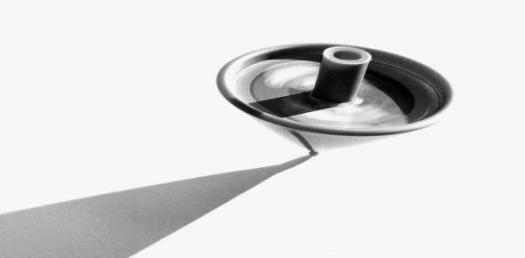# Angular Momentum Trivia Quiz

10 Questions | Total Attempts: 201SettingsAngular momentum is a pseudovector that represents the product of a body's rotational inertia and rotational velocity about a particular axis. Angular momentum depends on where the origin is chosen since the particle's position is measured from it. The total sum of the angular momentum of any composite system is the pseudovector sum of the angular momenta of its constituent parts. Try this quiz to test your knowledge on this topic.

• 1.
What is the common symbol for Angular momentum?
• A.

L

• B.

Q

• C.

A

• D.

M

• 2.
What is the dimension of Angular momentum?
• A.

ML²/T

• B.

ML/T

• C.

MLT²

• D.

ML²T²

• 3.
What is the relationship between orbital angular momentum and orbital angular velocity?
• A.

Directly Proportional

• B.

Inversely proportional

• C.

Jointly proportional

• D.

Perpendicular to each other

• 4.
Which of these denotes orbital angular velocity?
• A.

μ

• B.

Ω

• C.

ω

• D.

π

• 5.
How many types of angular momentum are there?
• A.

4

• B.

2

• C.

3

• D.

5

• 6.
Which of these is defined as the angular momentum about its centre of mass coordinate?
• A.

Spin angular momentum

• B.

Orbital angular momentum

• C.

• D.

Jouste angular momentum

• 7.
Which of these is defined as the angular momentum of the centre of mass about the origin?
• A.

Spin angular momentum

• B.

Orbital angular momentum

• C.

• D.

Jouste angular momentum

• 8.
What is the total angular momentum of an object?
• A.

Sum of Spin and Jouste momenta

• B.

Sum of orbital and radial momenta

• C.

Sum of radial and Jouste momenta

• D.

Sum of Spin and orbital momenta

• 9.
Which of these denotes spin angular velocity?Ω^
• A.

Π

• B.

μ

• C.

Ω

• D.

¢

• 10.
Which of these is defined as the rate of change of angular momentum, analogous to force?
• A.

Angular velocity

• B.Back to top